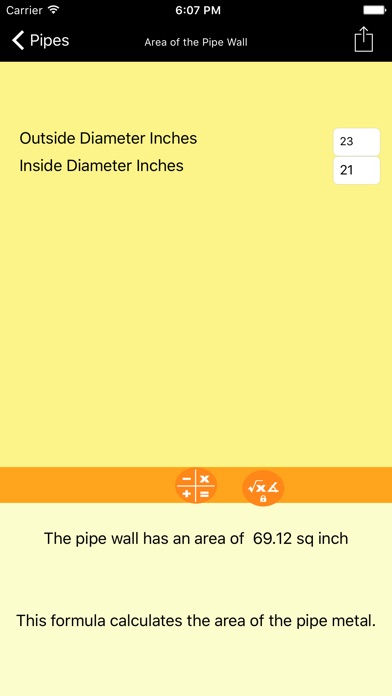Plumbing Formulator

iOS UniversalProductivity

Free

PLUMBING FORMULATOR contains over 120 formulas that are useful for plumbers and HVAC installers. The program calculates areas of pipe walls. It calculates the weights of different pipes. PLUMBING FORMULATOR also calculates the final temperature when two bodies of water are mixed. It calculates expansion of pipes, radiant heat and heat loss. The Plumbing Formulator has various formulas to calculate area and volumes of differing shapes. The program also includes dozens of conversions from everything from square feet to square inches to pounds square inches to feet of water.

Every result in PLUMBING FORMULATOR can be Emailed and printed. You can also select from favorites and recent formulas. In the coming months there will be program updates that will include additional formulas. If there is a formulas you would like that is not included just e-mail us and we will try to include them in the next update.

Some of the Formulas include:

PUMPS
• Pipe Velocity
• Water Horsepower
• Brake Horsepower –Pump
• Efficiency of Pump
• Brake Horsepower Motor
• Pressure

HVAC
• Length of Pipe
• Heat Loss
• Building Heat Loss
• Fresh Air in Room
• Water Chilling load
• Cooling Load in Ls/Tons
• Coefficient of Performance
• Water Required
• Final Temperature When Water is Mixed

PIPING
• Area of Pipe Wall
• Weight of Cast Iron Pipe
• Weight of Steel Pipe
• Weight of Copper Pipe
• Expansion in Plastic Piping
• Hazen William Friction Loss
• Pressure of Pipe
• Piping Elbows
• Piping Gate Valves
• Pipe Length Change Due to Temperature
• Chase Width
• Chase Depth
• Total Fall
• 45 Degree Offset

WATER FLOW
• Optimum Water Flow
• Volume of Water Flow
• Velocity of Water
• Pipes Total Dimension
• Supply Water Rate

WATER HEATERS
• BTU input
• Gallons Per Hour of Gas
• Domestic Daily Hot Water Usage

CHARTS
• Dozens of Plumbing Charts
BOOK
How to built in book on plumbing design

What's New

Bug fixes and optimized for iPhone X

•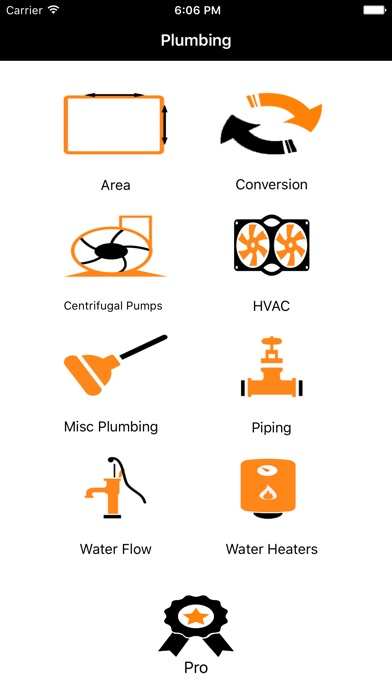•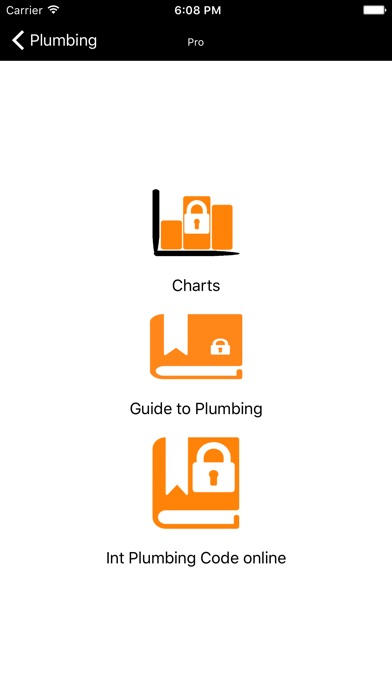•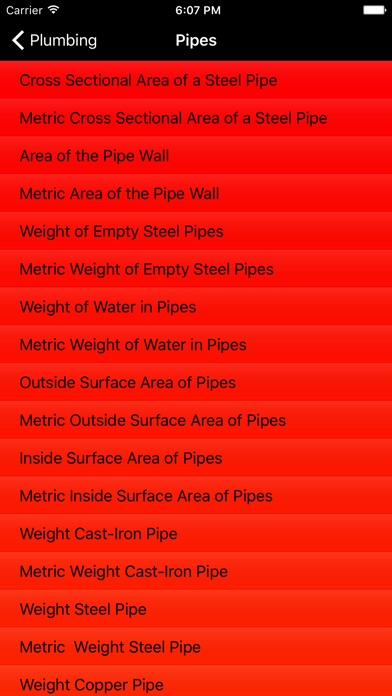•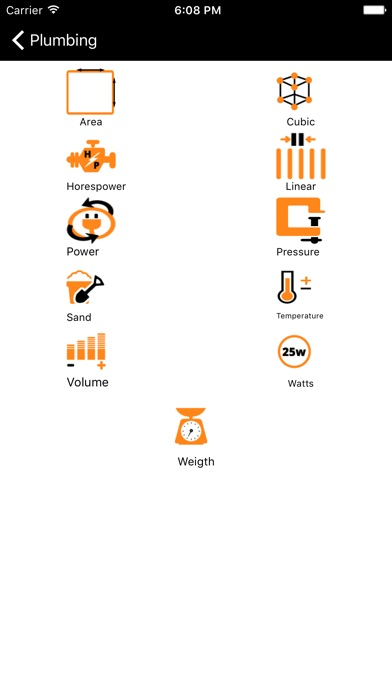•